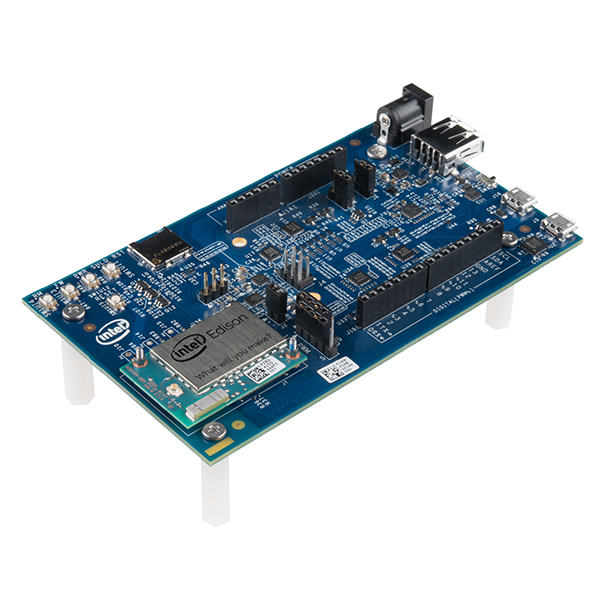Using Johnny-Five with Grove's RGB LCD component on the Intel Edison Arduino Breakout. This shield and component will work with any Arduino pin-out compatible hardware platform.

Run this example from the command line with:

``````node eg/grove-lcd-rgb-edison.js
``````
``````var five = require("johnny-five");
var Edison = require("edison-io");
var board = new five.Board({
io: new Edison()
});

// Plug the Rotary Angle sensor module
// into the Grove Shield's A0 jack
var rotary = new five.Sensor("A0");

// Plug the LCD module into any of the
// Grove Shield's I2C jacks.
var lcd = new five.LCD({
controller: "JHD1313M1"
});

// Set scaling of the Rotary angle
// sensor's output to 0-255 (8-bit)
// range. Set the LCD's background
// color to a RGB value between
// Red and Violet based on the
// value of the rotary sensor.
rotary.scale(0, 255).on("change", function() {
var r = linear(0xFF, 0x4B, this.value, 0xFF);
var g = linear(0x00, 0x00, this.value, 0xFF);
var b = linear(0x00, 0x82, this.value, 0xFF);

lcd.bgColor(r, g, b);
});
});

// [Linear Interpolation](https://en.wikipedia.org/wiki/Linear_interpolation)
function linear(start, end, step, steps) {
return (end - start) * step / steps + start;
}

``````

For this program, you'll need: